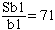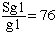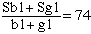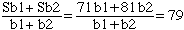Name: rajesh Who is asking: Other Level: Secondary Question: The students of two schools were sitting the same test. The students attending the first school scored 74 on average; within this the average scores of the boys and the girls were 71 and 76, respectively. The corresponding scores in the other school were 84, 81 and 90, respectively. If the average score of all boys was 79, what did the girls score on average?? i am not able to crack this Hi Rajesh, Look at the first school. Suppose that the number of boys in the first school is b1, the number of girls is g1, the sum of the grades for the boys is Sb1 and the sum of the grades for the girls is Sg1. Since the average grade of the boys is 71,and since the average grade for the girls is 76,Hence Sb1 = 71 b1 and Sg1 = 76 g1. The average grade of all the students in the first school is 74 and hence,or 71 b1 + 76 g1 = 74(b1 + g1) which simplifies to 2 g1 = 3 b1 Using analogous notation for the second school you can see that 2 g2 = b2 In a similar fashion the average for the boys iswhich leads to b2 = 4 b1. Finally write an expression for the average grade of the girls, use the relations you have between b1, b2, g1 and g2 to write this expression in terms of b1 only, and then simplify. Cheers, Penny Go to Math Central